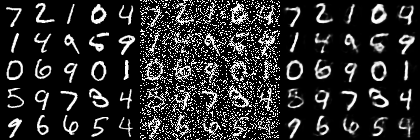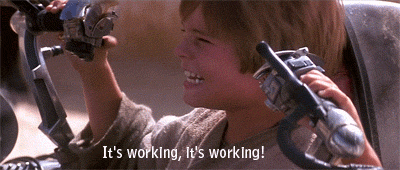# Tutorial: Your First Model (DAE)

Write your own denoising autoencoder and train it on MNIST

If you followed Tutorial: First Steps, you learned how to train and use an existing model on a dataset. In this tutorial, you will see how easy it is to implement your own models in OpenDeep.

Most other places will have you start with a traditional feed-forward Multilayer Perceptron, but we know you are better than that! Let's implement something cool like an unsupervised denoising autoencoder and use it to reconstruct MNIST. Then in another tutorial will show you how to make it into a supervised classifier.

Before you begin, you should be familiar with Theano, especially with the basics in baby steps - algebra for creating variables and functions. It is also a good idea to set up Theano to use the GPU to reduce training time.

I also recommend knowing the basics of how autoencoders work. There is already a great tutorial in Theano for denoising autoencoders here: Denoising Autoencoder.

In this tutorial, we are going to start with the bare minimum for creating a denoising autoencoder to work with MNIST data, and then show how to make it flexible in the OpenDeep style!

# Creating a bare-bones Model

The Model class in OpenDeep provides an interface for the functionality a model needs to work with Datasets and Optimizers. At a minimum to be useful, a model needs to be able to train and predict. This means you only need to write four methods. Yes, you heard right, only four methods:

• get_inputs(): return a list of the inputs your model accepts. In our case, a single matrix.
• get_params(): return a list of the model parameters. In our case, a weights matrix and a bias vector.
• get_train_cost(): return an expression of the training cost for the model.
• get_outputs(): return a Theano expression for computing outputs given inputs - the whole point of having a model!

Let's jump right in!
#Initializing a denoising autoencoder
During initialization, you can set up the hyperparameters and perform the main computations for your model (not best practice to be monolithic like this, but you can get away with it for personal research).

``````import theano.tensor as T
from opendeep.models.model import Model
from opendeep.utils.nnet import get_weights_uniform, get_bias
from opendeep.utils.noise import salt_and_pepper
from opendeep.utils.activation import tanh, sigmoid
from opendeep.utils.cost import binary_crossentropy

# create our class initialization!
class DenoisingAutoencoder(Model):
"""
A denoising autoencoder will corrupt an input (add noise) and try to reconstruct it.
"""
def __init__(self):
# Define some model hyperparameters to work with MNIST images!
input_size  = 28*28 # dimensions of image
hidden_size = 1000  # number of hidden units - generally bigger than input size for DAE

# Now, define the symbolic input to the model (Theano)
# We use a matrix rather than a vector so that minibatch processing can be done in parallel.
x = T.fmatrix("X")
self.inputs = [x]

# Build the model's parameters - a weight matrix and two bias vectors
W  = get_weights_uniform(shape=(input_size, hidden_size), name="W")
b0 = get_bias(shape=input_size, name="b0")
b1 = get_bias(shape=hidden_size, name="b1")
self.params = [W, b0, b1]

# Perform the computation for a denoising autoencoder!
# first, add noise (corrupt) the input
corrupted_input = salt_and_pepper(input=x, corruption_level=0.4)
# next, compute the hidden layer given the inputs (the encoding function)
hiddens = tanh(T.dot(corrupted_input, W) + b1)
# finally, create the reconstruction from the hidden layer (we tie the weights with W.T)
reconstruction = sigmoid(T.dot(hiddens, W.T) + b0)
# the training cost is reconstruction error - with MNIST this is binary cross-entropy
self.train_cost = binary_crossentropy(output=reconstruction, target=x)

# Compile everything into a Theano function for prediction!
# When using real-world data in predictions, we wouldn't corrupt the input first.
# Therefore, create another version of the hiddens and reconstruction without adding the noise
hiddens_predict = tanh(T.dot(x, W) + b1)
recon_predict   = sigmoid(T.dot(hiddens_predict, W.T) + b0)
self.output     = recon_predict
``````

Then all we need to do is add the four methods listed above. Because we created the model's computational logic in the `__init__` method, this is as simple as returning the variables. See below:

``````def get_inputs(self):
return self.inputs

def get_params(self):
return self.params

def get_train_cost(self):
return self.train_cost

def get_outputs(self):
return self.output
``````

That was easy! Now, we can train and evaluate our shiny new model. To put everything together, here is the completed class with code to run on MNIST:

``````import theano.tensor as T
from opendeep.models.model import Model
from opendeep.utils.nnet import get_weights_uniform, get_bias
from opendeep.utils.noise import salt_and_pepper
from opendeep.utils.activation import tanh, sigmoid
from opendeep.utils.cost import binary_crossentropy

# create our class initialization!
class DenoisingAutoencoder(Model):
"""
A denoising autoencoder will corrupt an input (add noise) and try to reconstruct it.
"""
def __init__(self):
# Define some model hyperparameters to work with MNIST images!
input_size  = 28*28 # dimensions of image
hidden_size = 1000  # number of hidden units - generally bigger than input size for DAE

# Now, define the symbolic input to the model (Theano)
# We use a matrix rather than a vector so that minibatch processing can be done in parallel.
x = T.fmatrix("X")
self.inputs = [x]

# Build the model's parameters - a weight matrix and two bias vectors
W  = get_weights_uniform(shape=(input_size, hidden_size), name="W")
b0 = get_bias(shape=input_size, name="b0")
b1 = get_bias(shape=hidden_size, name="b1")
self.params = [W, b0, b1]

# Perform the computation for a denoising autoencoder!
# first, add noise (corrupt) the input
corrupted_input = salt_and_pepper(input=x, corruption_level=0.4)
# next, compute the hidden layer given the inputs (the encoding function)
hiddens = tanh(T.dot(corrupted_input, W) + b1)
# finally, create the reconstruction from the hidden layer (we tie the weights with W.T)
reconstruction = sigmoid(T.dot(hiddens, W.T) + b0)
# the training cost is reconstruction error - with MNIST this is binary cross-entropy
self.train_cost = binary_crossentropy(output=reconstruction, target=x)

# Compile everything into a Theano function for prediction!
# When using real-world data in predictions, we wouldn't corrupt the input first.
# Therefore, create another version of the hiddens and reconstruction without adding the noise
hiddens_predict = tanh(T.dot(x, W) + b1)
recon_predict   = sigmoid(T.dot(hiddens_predict, W.T) + b0)
self.output       = recon_predict

def get_inputs(self):
return self.inputs

def get_params(self):
return self.params

def get_train_cost(self):
return self.train_cost

def get_outputs(self):
return self.output

if __name__ == '__main__':
# set up the logging environment to display outputs (optional)
# although this is recommended over print statements everywhere
import logging
from opendeep.log.logger import config_root_logger
config_root_logger()
log = logging.getLogger(__name__)
log.info("Creating a Denoising Autoencoder!")

# import the dataset and optimizer to use
from opendeep.data.dataset import TEST
from opendeep.data.standard_datasets.image.mnist import MNIST

# grab the MNIST dataset
mnist = MNIST()

# create your shiny new DAE
dae = DenoisingAutoencoder()

# make an optimizer to train it (AdaDelta is a good default)
# perform training!
optimizer.train()

# test it on some images!
test_data = mnist.getDataByIndices(indices=range(25), subset=TEST)
corrupted_test = salt_and_pepper(test_data, 0.4).eval()
# use the predict function!
reconstructed_images = dae.predict(corrupted_test)

# create an image from this reconstruction!
# imports for working with tiling outputs into one image
from opendeep.utils.image import tile_raster_images
import numpy
import PIL
# stack the image matrices together in three 5x5 grids next to each other using numpy
stacked = numpy.vstack(
[numpy.vstack([test_data[i*5 : (i+1)*5],
corrupted_test[i*5 : (i+1)*5],
reconstructed_images[i*5 : (i+1)*5]])
for i in range(5)])
# convert the combined matrix into an image
image = PIL.Image.fromarray(
tile_raster_images(stacked, (28, 28), (5, 3*5))
)
# save it!
image.save("dae_reconstruction_test.png")
``````

Huzzah! This code should produce an image that looks like this after ~350 training epochs:Left: original test images. Center: corrupted (noisy) images. Right: reconstructed images (output).

Congrats, you just created a brand new unsupervised model!